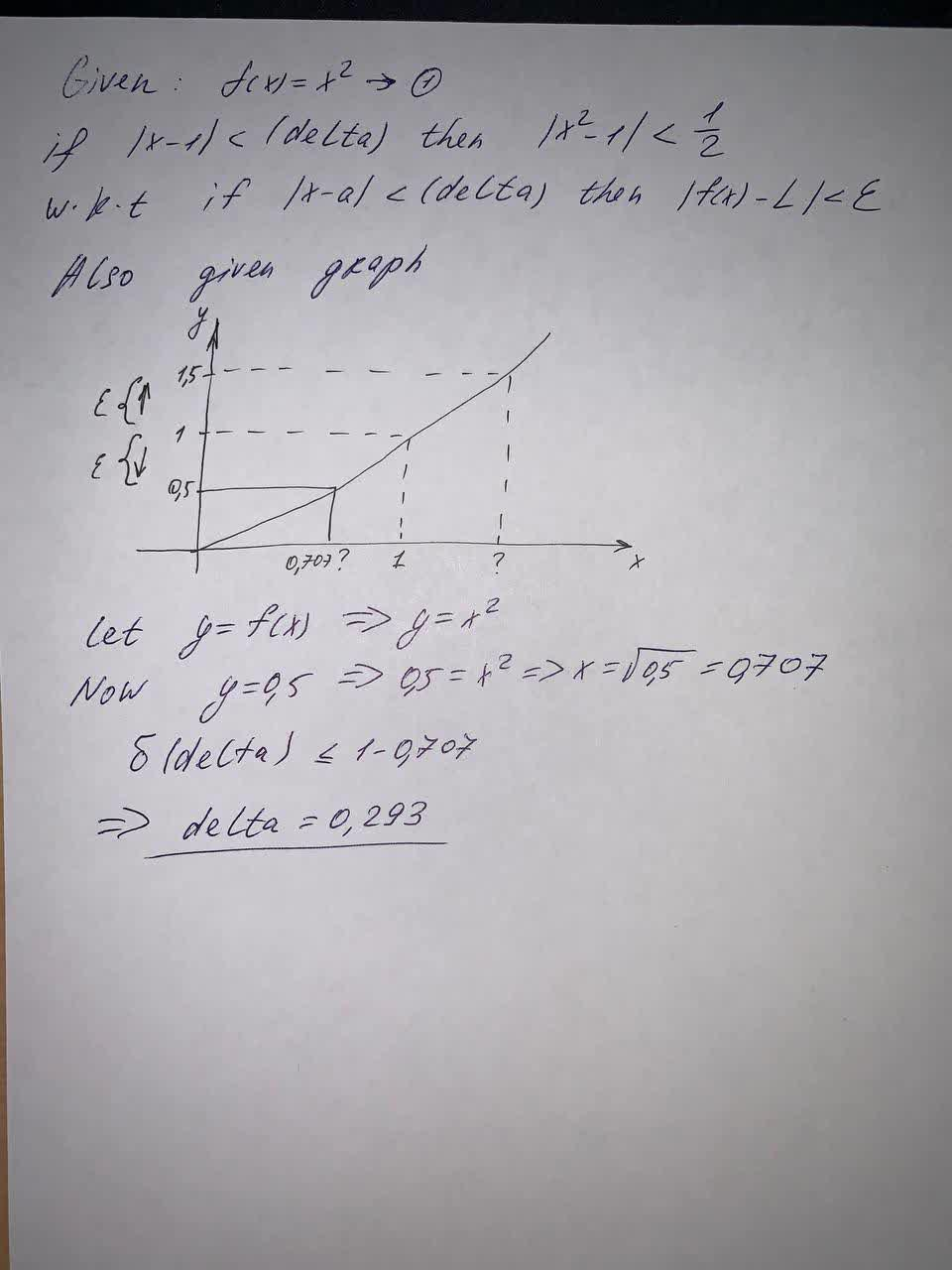Use the given graph off(x) = x^2to find a number (delta) such thatif |x - 1| < (delta) then |x^2-1| < \frac{1}{2}(Round your answer down to three decimal places.)(delta) =1210500781.jpgZoe Oneal 2021-06-10 Answered

Use the given graph of
$$f(x) = x^2$$
to find a number $$(\delta)$$ such that
if $$|x - 1| < (\delta)$$ then $$|x^2-1| < \frac{1}{2}$$
$$(\delta) =$$

• Questions are typically answered in as fast as 30 minutes

Solve your problem for the price of one coffee

• Math expert for every subject
• Pay only if we can solve itunessodopunsep